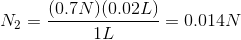# MCAT Physical : Concentration, Dilution, and Units

## Example Questions

### Example Question #1 : Concentration, Dilution, And Units

What is the molarity of a 1L solution composed of water and 300g of sodium iodide?

4M

2M

0.5M

1M

2M

Explanation:

Sodium iodide is given by the formula NaI, and has a molecular weight of 150g/mol. Molarity is found by dividing the moles of solute by liters of solvent.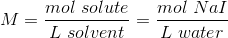We find the moles of sodium iodide by using the mass (300g) and molecular weight.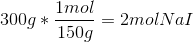We know our volume is 1L, so now we can solve for the molarity.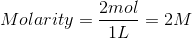### Example Question #2 : Concentration, Dilution, And Units

What is the final concentration of 100mL of 6M HCl solution when it is diluted with 500mL of water?

10M

0.6M

1.2M

1M

1M

Explanation:

To solve this question we must first determine the number of moles of HCl in the initial solution. Converting 100mL into 0.1L, there is initially 0.6mol of HCl in the solution.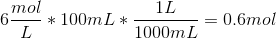When we dilute the solution with 500mL of water, the final volume of solution is 600mL.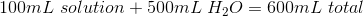To find molarity we can divide number of moles of solute by the total volume of solution.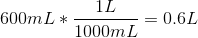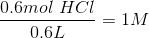The final concentration is 1M.

### Example Question #1 : Concentration, Dilution, And Units

What is the molality of a solution when 300mmol of HBr is added to 60g of ether?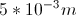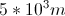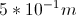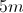Explanation:

Molality is the number of moles of solute divided by kilograms of solvent. Because we are given the solute in mmol and the solvent in grams, we must convert them to moles and kilograms, respectively.

300 mmol is 0.3 moles.  Additionally 60 grams is 6 x 10-2 kg.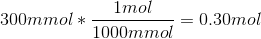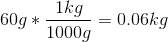Now we can solve for the molality.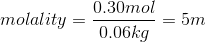### Example Question #1 : Concentration, Dilution, And Units

Which of the following aqueous solutions is the most concentrated?

2M

20 grams of ammonia mixed with 1kg of water

20 grams of ammonia mixed to make 1 liter of solution

2m

2M

Explanation:

20 grams of ammonia is approximately 1.17 moles of ammonia, so both the molarity and molality of this solution would not approach 2.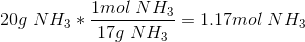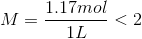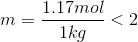Now, we need to determine whether molarity or molality is more concentrated. It helps to remember for the MCAT that 1 liter of water is equal to 1 kilogram of water. Molality uses the denomination of kilograms of water, and molarity mixes the solute until liters of solution are created. Since the mixed solution will incorporate the solute, it will require less than 1 kilogram of water. As a result, 2M is more concentrated than 2m.

### Example Question #5 : Concentration, Dilution, And Units

A lab technician prepares a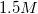aqueous solution of copper (II) sulfate. The solution has a density of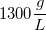. What is the ratio of moles of copper (II) sulfate to moles of water in this solution?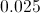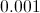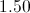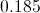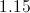Explanation:

First, find the mass of copper (II) sulfate in one liter of solution, using the molar mass of copper (II) sulfate.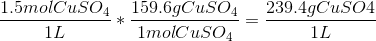We now have the density of the copper (II) sulfate in the solution. Using the total density of the solution, we can calculate the contribution from water to this density.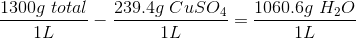Now that we know the mass of water per liter of solution, we need to convert to moles.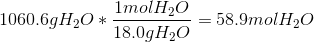We now have the moles of copper (II) sulfate per liter and the moles of water per liter, allowing us to find the molar ratio in the solution.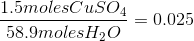### Example Question #6 : Concentration, Dilution, And Units

What is the mole fraction of sucrose (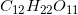) in a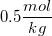solution?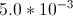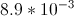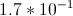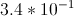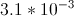Explanation:

Recall that molality is defined as:In this problem there is one half mole of sucrose per one kilogram of water.

Our goal is to calculate the mole fraction: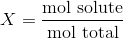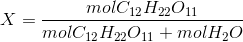We need to calculate the number of moles of water in one kilogram of solution.We now know that, in one kilogram of solution, there will be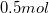of sucrose and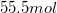of water. Use these values in the mole fraction equation to solve for the fraction of sucrose.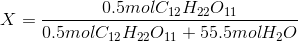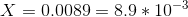### Example Question #7 : Concentration, Dilution, And Units

Which of the following is true of a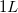sample of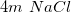solution?

It contains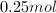of sodium chloride

It contains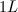of water

It is equal in concentration to a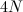solution of sodium chloride

It is equal in concentration to a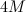solution of sodium chloride

It contains less water than asample of asolution of sodium chloride

It contains less water than asample of asolution of sodium chloride

Explanation:

Concentration can be measured in several different ways. Molality is moles of solute per kilogram of solvent. Molarity is moles of solute per liter of solution. Normality is equivalents of protons per liter of solution.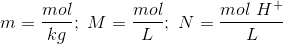In our question, we are given the molality of the solution.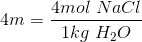When using molality, it is important to realize that the solute occupies volume. One kilogram of water is equal to one liter of water, but there is an additional volume of four moles of solid sodium chloride that is added to the solution. A one-liter sample of this solution will, thus, contain less than one liter of water.

### Example Question #8 : Concentration, Dilution, And Units

A chemist has 300mL of a 0.75M solution and needs to dilute it to 0.35M. How much solvent should be added?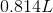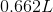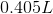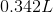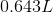Explanation:

Use the equation for molarity,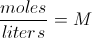, first to figure out the number of moles of solute present.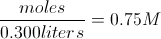Solving this gives 0.225 moles of solute. Using the same equation again with the final molarity will give the final volume.Finally, the amount of solvent added is the difference between initial and final total volumes.

0.643L – 0.300L = 0.342L added

### Example Question #71 : Solution Chemistry

If 0.02L of a 0.7N NaCl solution is diluted to 1L, what is the new concentration?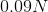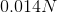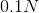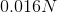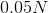Explanation:

In this particular scenario, molarity can be used interchangeably with normality. The product of the initial normality and initial volume will be equal to the product of the final normality and final volume.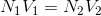Our initial normality is 0.7N and our initial volume is 0.02L. We also know our final volume is 1L.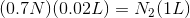Solve for the final concentration.